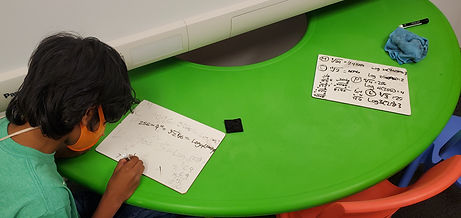Mr. Jake

Target 1​

Lesson Type:

Continuation

Number Operation

:

Computation

Solve arithmetic expressions that include exponents.

1:

Determine the exponential rules or laws that apply to multiplication and division.

2:

Solve expressions using exponential rules and laws.

3:

Determine that there are no “short-cuts” for the addition and subtraction of exponential expressions (no matter what the bases or exponents are).

4:

Use the product rule to multiply expressions with like bases (ax * ay = ax+y).

5:

Use the quotient rule to divide expression with like bases (ax ÷ ay = ax-y).

7th

Vocabulary:

Exponent, Logarithms, Root

Activities:

Practiced solving equations that include exponents Introduced how to write exponents in root and logarithmic formHome Exploration

Guiding Questions:Absent Students:

Target 2

:

1:

Look at a visual spatial puzzle to analyze the pieces and determine which is the correct piece to choose.

2:

Look at a visual puzzle to analyze the pieces and determine which is the correct piece to choose.

6th

Vocabulary:

Tower of hanoi

Activities:

Solved tower of Hanoi puzzles

Determined the minimal number of moves needed to solve the different forms of the tower of Hanoi puzzleHome Exploration

Guiding Questions:Target 3

:

Vocabulary:

Activities:Home Exploration